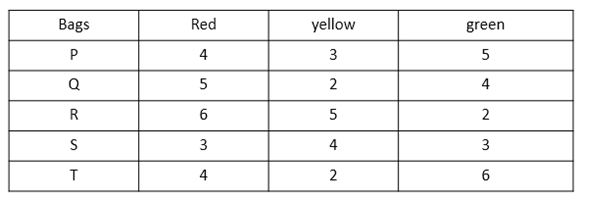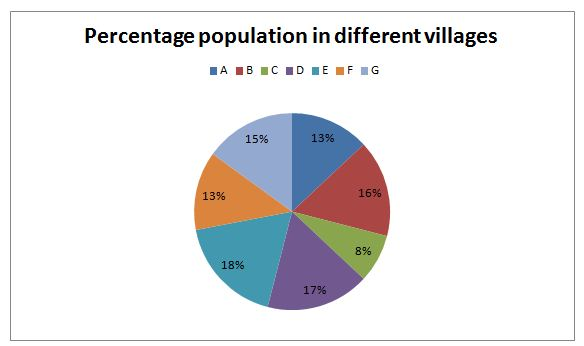# Quantitative Aptitude Questions (Data Interpretation) for SBI Clerk Mains 2018 Day-169

Dear Readers, SBI is conducting Online Examination for the recruitment of clerical cadre To enrich your preparation here we have providing new series of Data Interpretation – Quantitative Aptitude Questions. Candidates those who are appearing in SBI Clerk Mains Exams can practice these Quantitative Aptitude average questions daily and make your preparation effective.

[WpProQuiz 3137]

Click “Start Quiz” to attend these Questions and view Solutions

Directions (Q. 1 – 5): Study the following information carefully and answer the questions given questions.

Following table shows the different color of balls in 5 different bags.1) If three balls are drawn at random from bag Q, then find the probability that all the balls are of different colors?

a) 3/11

b) 4/33

c) 8/33

d) 4/11

e) None of these

2) Total number of red balls and yellow balls together is what percent of total number of green balls?

a) 150 %

b) 160 %

c) 180 %

d) 190 %

e) 170 %

3) If four balls are drawn at random from bag R, then find the probability of taking at least one yellow ball?

a) 14/143

b) 129/143

c) 27/143

d) 15/143

e) None of these

4) If three balls are drawn from each of the bags S and T, then find the ratio of that out of three balls 2 are yellow from bag S and all the balls from bag T are green?

a) 11 : 10

b) 33 : 10

c) 43 : 11

d) 35 : 33

e) None of these

5) If three balls are drawn from both the bags P and R, then find the product of their probabilities that all the balls drawn from both the bags are of different colors?

a) 90/1573

b) 90/1373

c) 99/1573

d) 90/1373

e) None of these

Directions (Q. 6 – 10): Study the following information carefully and answer the given questions.

Following pie Chart shows the percentage of population in seven villages in 2006.The table below shows percentage of population below poverty line in every village:

 Villages Percentage of people below poverty line Ratio of Male to Female A 45 % 6 : 7 B 50 % 1 : 2 C 60 % 5 : 7 D 40 % 12 : 13 E 20 % 7 : 5 F 25 % 1 : 1 G 30 % 3 : 5

6) If the total population of all the villages in 2006 is 120 lakhs, then find the difference between the number of people who are above poverty line in villages A and C?

a) 4.74 lakhs

b) 5.70 lakhs

c) 2.87 lakhs

d) 5.44 lakhs

e) None of these

7) If the number of people below poverty line in village G is 9 lakhs, then find the difference between the number of males and females in village E?

a) 8 lakhs

b) 10 lakhs

c) 6 lakhs

d) 12 lakhs

e) None of these

8) If the male population of village F in 2007 is 10 lakhs which is 150 % more than the male population of village F in 2006, then find the number of people above poverty level in village F in 2006?

a) 12 lakhs

b) 8 lakhs

c) 15 lakhs

d) 6 lakhs

e) None of these

9) If the total population of village B and D is 99 lakhs, then find the difference between the male population of village C and female population of village A?

a) 7 lakhs

b) 15 lakhs

c) 18 lakhs

d) 11 lakhs

e) None of these

10) If the number of people below poverty level in village G is 18 lakhs, then find the male population of village E is what percentage more than the female population of village E?

a) 20

b) 12

c) 20

d) 40

e) None of these

Direction (1-5)

Required probability = (5c1 x 2c1 x 4c1)/11c3

= > (5 x 2 x 4)/165 = 8/33

Total number of red balls = 4 + 5 + 6 + 3 + 4 = 22

Total number of yellow balls = 3 + 2 + 5 + 4 + 2 = 16

Total number of green balls = 5 + 4 + 2 + 3 + 6 = 20

Required % = [(22 + 16)/20]*100 = 190 %

Probability of taking at least one yellow ball = 1 – P(none is yellow ball)

P(None is yellow ball)

= > 8C4/13C4

= > 14/143

Probability of taking at least one yellow ball = 1 – (14/143) = 129/143

Probability that out of 3 balls from bag S, 2 are yellow

= > (4c2 x 6c1)/10c3 = 36/ 120 = 3/10

Probability that all the balls from bag T are green = 6c3/12c3 = 1/11

Required ratio = (3/10) : (1/11) = 33 : 10

Probability of all the balls drawn from bag P are of different colors

= (4c1 x 3c1 x 5c1)/12c3

= (4 x 3 x 5)/220

= 3/11

Probability of all the balls drawn from bag R are of different colors

= (6c1 x 5c1 x 2c1)/13c3

= (6 x 5 x 2)/286

= 30/143

Required product = (3/11)*(30/143) = 90/1573

Direction (6-10)

Population of village A above poverty line

= > 120*(13/100)*(55/100) = 8.58 lakhs

Population of village C above poverty line

= > 120*(8/100)*(40/100) = 3.84 lakhs

Required difference = 8.58 – 3.84 = 4.74 lakhs

(30/100)* Total population of village G = 9 lakhs

Total population of village G = (9/30)*100= 30 lakhs

Total population of all the villages = (30/15)*100= 200 lakhs

Difference between number of males and females in village E

= > 200*(18/100)*(2/12) = 6 lakhs

Male population of village F in 2006 = (10/250)*100 = 4 lakhs

Total population of village F in 2006 = 4*2 = 8 lakhs

The number of people above poverty level in Village F in 2006

= > 8*(75/100) = 6 lakhs

Total population of all the villages = (99/33)*100 = 300 lakhs

Male population in village C = (8/100)*300*(5/12) = 10 lakhs

Female population in village A= (13/100)*300*(7/13) = 21 lakhs

Required difference = 21 – 10 = 11 lakhs

Total population of Village G = (18/30)*100 = 60 lakhs

Total population of all the village = (60/15)*100 = 400 lakhs

Male population of village E = 400*(18/100)*(7/12) = 42 lakhs

Female population of village E = 400*(18/100)*(5/12) = 30 lakhs

Required percentage = [(42 – 30)/30]*100 = 40%

Daily Practice Test Schedule | Good Luck

 Topic Daily Publishing Time Daily News Papers & Editorials 8.00 AM Current Affairs Quiz 9.00 AM Quantitative Aptitude “20-20” 11.00 AM Vocabulary (Based on The Hindu) 12.00 PM General Awareness “20-20” 1.00 PM English Language “20-20” 2.00 PM Reasoning Puzzles & Seating 4.00 PM Daily Current Affairs Updates 5.00 PM Data Interpretation / Application Sums (Topic Wise) 6.00 PM Reasoning Ability “20-20” 7.00 PM English Language (New Pattern Questions) 8.00 PM